Custom SearchDISTANCE FROM A POINT TO A LINE We must often express the distance from a point to a line in terms of the coefficients in the equation of the line. To do this, we compare the two forms of the equation of a straight line, as follows: General equation: Ax + By + C = 0 Normal form: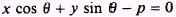The general equation and the normal form represent the same straight line. Therefore, A (the coefficient of x in the general form) is proportional to cos 0 (the coefficient of x in the normal form). By similar reasoning, B is proportional to sin 0, and C is proportional to -p. Recalling that quantities proportional to each other form ratios involving a constant of proportionality, let k be this constant. Thus, we have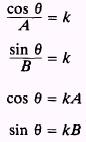Squaring both sides of these two expressions and then adding, we have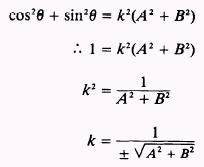The coefficients in the normal form, expressed in terms of A, B, and C, are as follows: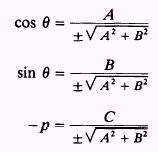The sign of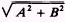is chosen so as to make p (a distance) always positive. The conversion formulas developed in the foregoing discussion are used in finding the distance from a point to a line. Let p represent the distance of line LK from the origin. (See fig. 1-10.) To find d, the distance from point P, to line LK, we construct a lineFigure 1-10.-Distance from a point to a line. through P, parallel to LK. The distance of this line from the origin is OS, and the difference between OS and p is d. We obtain an expression for d, based on the coordinates of P,, as follows: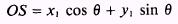and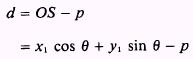Returning to the expressions for sin 0, cos 0, and p in terms of A, B, and C (the coefficients in the general equation), we have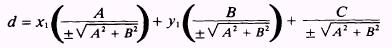In the formula for d, the denominator in each of the expressions is the same. Therefore, we may combine terms as follows:We use the absolute value, since d is a distance, and thus avoid any confusion arising from the radical. Note that the absolute value,|| of a number is defined as follows: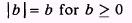andThat is, for the positive number 2, |2|=2 For the negative number -2,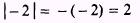The absolute value of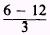is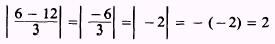EXAMPLE: Find the distance from the point (2,1) to the line 4x+2y+7=0. SOLUTION: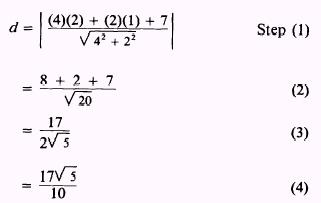PRACTICE PROBLEMS: In each of the following problems point to the line: find the distance from the 1. (5,2), 3x - y + 6 = 0 2. (-2,5), 3x + 4y - 9 = 0 ANSWERS: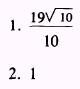SUMMARY The following are the major topics covered in this chapter: 1. Distance between two points:where (x,,y,) and (x2,y2) are given points on a line. 2. Division of a line segment: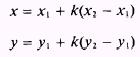where k is the desired proportion of the distance between points (x,,y) and (x2,y2) and (x,y) is the desired point. 3. Midpoint of a line segment:4. Inclination: The the angle the line crossing the X axis makes with the positively directed portion of the X axis, such that 0 < a < 180'. 5. Slope: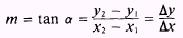where e means "change in." The slope of a horizontal line is zero. The slope of a vertical line is meaningless. 6. Slopes of parallel lines: Slopes are equal or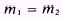where m, and m2 are the slopes of the lines L, and L2, respectively. 7. Slopes of perpendicular lines: Slopes are negative reciprocals or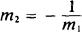8. Acute angle between two lines:However, if one line, L2, is parallel to the Y axis and the other, L,, has a positive slope, then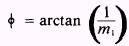If L2 is parallel to the Y axis and L, has a negative slope, then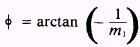9. Obtuse angle between two lines: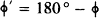where + is the acute angle between the two lines. 10. Point-slope form of a straight line: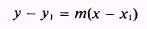11. Slope-intercept form of a straight line: y=mx+b where b is the y intercept. 12. Normal form of a straight line: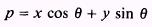where p is the line's perpendicular distance from the origin and 0 is the angle between the perpendicular and the X axis. 13. Parallel lines: In any two linear equations, if the coefficients of the x and y terms are identical in value and sign, then the lines represented by these equations are parallel; that is, Ax+By+C=0 and Ax+By+D=0 are parallel lines. 14. Perpendicular lines: If a line is to be perpendicular to a given line, the coefficients of x and y in the required equations are found by interchanging the coefficients of x and y in the given equation and changing the sign of one of them; that is, Ax+By+C=0 and Bx-Ay+D=0 are perpendicular lines. 15. Distance from a point to a line: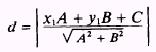where A, B, and C are the coefficients of the general equation of a line Ax + By + C = 0 and (x,,y,) are the coordinates of the point. ADDITIONAL PRACTICE PROBLEMS 1. Find the distance between P, (- 3, - 2) and P2(-7,I). 2. Find the distance between P, (- 3/4, - 2) and PZ(1, - 1/2). 3. Find the coordinates of a point 115 of the way from P,(- 2,0) to Pz(3, - 5). 4. Find the midpoint of the line between P,(-8/3,4/5) and P2(- 4/3,6/5). 5. Find the slope of the line joining P,(4,6) and Pz(-4,6). 6. Find the slope of the line parallel to the line joining P,(7,4) and Pz(4,7). 7. Find the slope of the line perpendicular to the line joining P,(8,1) and PZ(2,4). 8. Find the obtuse angle between the two lines which have m, = 7 and m2 = - 3 for slopes. 9. Find the obtuse angle between the Y axis and a line with a slope of m = -1/4. 10. Find the equation of the line through the points ( - 6,5) and (6,5). 11. Find the equation of the line whose y intercept is (0,0) and whose slope is 4. 12. Find the slope and y intercept of the line whose equation is 4y+8x=7. 13. Find the equation of the line that is 3/2 units away from the origin, if the perpendicular from the line to the origin forms an angle of 2100 from the positive side of the X axis. 14. Find the equation of the line through (2,3) and perpendicular to 3x-2y=7. 15. Find the equation of the line through (2,3) and parallel to 3x-2y=7. 16. Find the distance from the point (3, - 5) to the line 2x+y+4=0. 17. Find the distance from the point (3, - 4) to the line 4x +3y= 10. ANSWERS TO ADDITIONAL PRACTICE PROBLEMS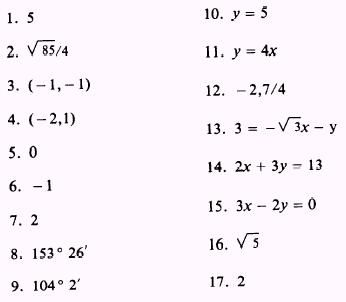Integrated Publishing, Inc. - A (SDVOSB) Service Disabled Veteran Owned Small Business